Next: 2.7 Assembling Up: 2. Finite Element Method Previous: 2.5 Finite Element Spaces

Subsections

# 2.6 Newton Methods

## 2.6.1 Introduction

The discretization of the non-linear PDE systems leads to a large system of nonlinear algebraic equations. There are various methods which solve such systems. Among them the most popular is a Newton method with it's variations.

## 2.6.2 Basic Newton Method

Generally we have a system of N non-linear algebraic equations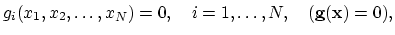(2.32)

assuming that the functions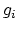have continues first order derivations with respect to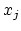. If the values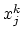of the-th approximate solution are known, we write Taylor series expansion of the functionsin the vicinity of,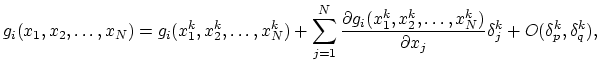(2.33)

for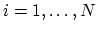, where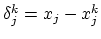. By choosing forthe exact value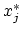and neglecting the terms of order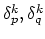we have from (2.33)(2.34)

The matrix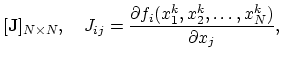(2.35)

is called Jacobi matrix. In the case when this matrix is non-singular the linear equation system (2.34) can be solved. Depending on the problem itself different types of linear solvers should be applied .
The solutions of the linear system (2.34) are the increments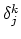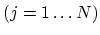and with these increments we can calculate new approximation,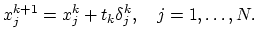(2.36)

The determination of damping value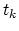is the question of trial and error. The sequences often used for subsequent trials are,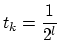or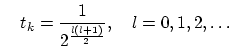(2.37)is accepted if the following criterion is fulfilled,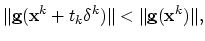(2.38)

where we use Euclidian norm.

## 2.6.3 Simplified Newton Method

Since the calculation of the Jacobi matrix is a very computer resources-consuming procedure, one can for a number of subsequent Newton iterations also use the same Jacobi matrix (2.35). In that case on obtains,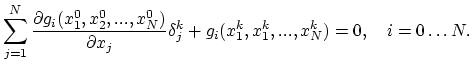(2.39)

The simplified Newton method obviously has a worse convergence behavior.

## 2.6.4 Damping

In the cases where the convergence of the Newton scheme is attainable only for a very small time steps, methods for the enforcing of the convergence must be applied. The method which has also successfully been applied in semiconductor device modelling , is the so-called Bank-Rose algorithm .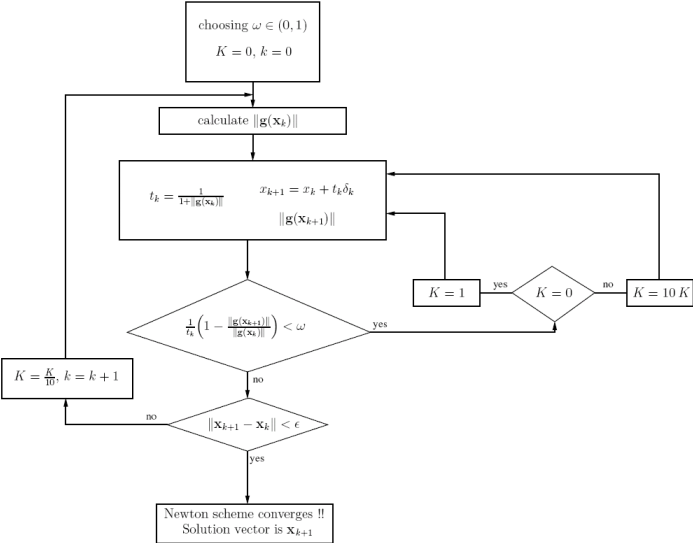The algorithm is represented in Figure 2.3. It controls the behavior of Newton scheme through a damping factor. The parameter of crucial importance for the algorithm is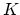. In Figure 2.3 we takeas initial estimate for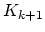. Alternatively, we have used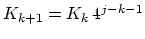, where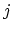is the counter of failed tests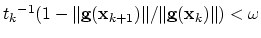.
The algorithm searchs through the vincinity of the initial solution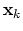for the shortest path through the attraction region of the Newton scheme to reach the next approximation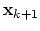. In the cases when the Bank-Rose algorithm fails the parametergrows infinitely and an improved approximationis never found.Next: 2.7 Assembling Up: 2. Finite Element Method Previous: 2.5 Finite Element Spaces

H. Ceric: Numerical Techniques in Modern TCAD